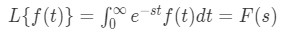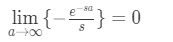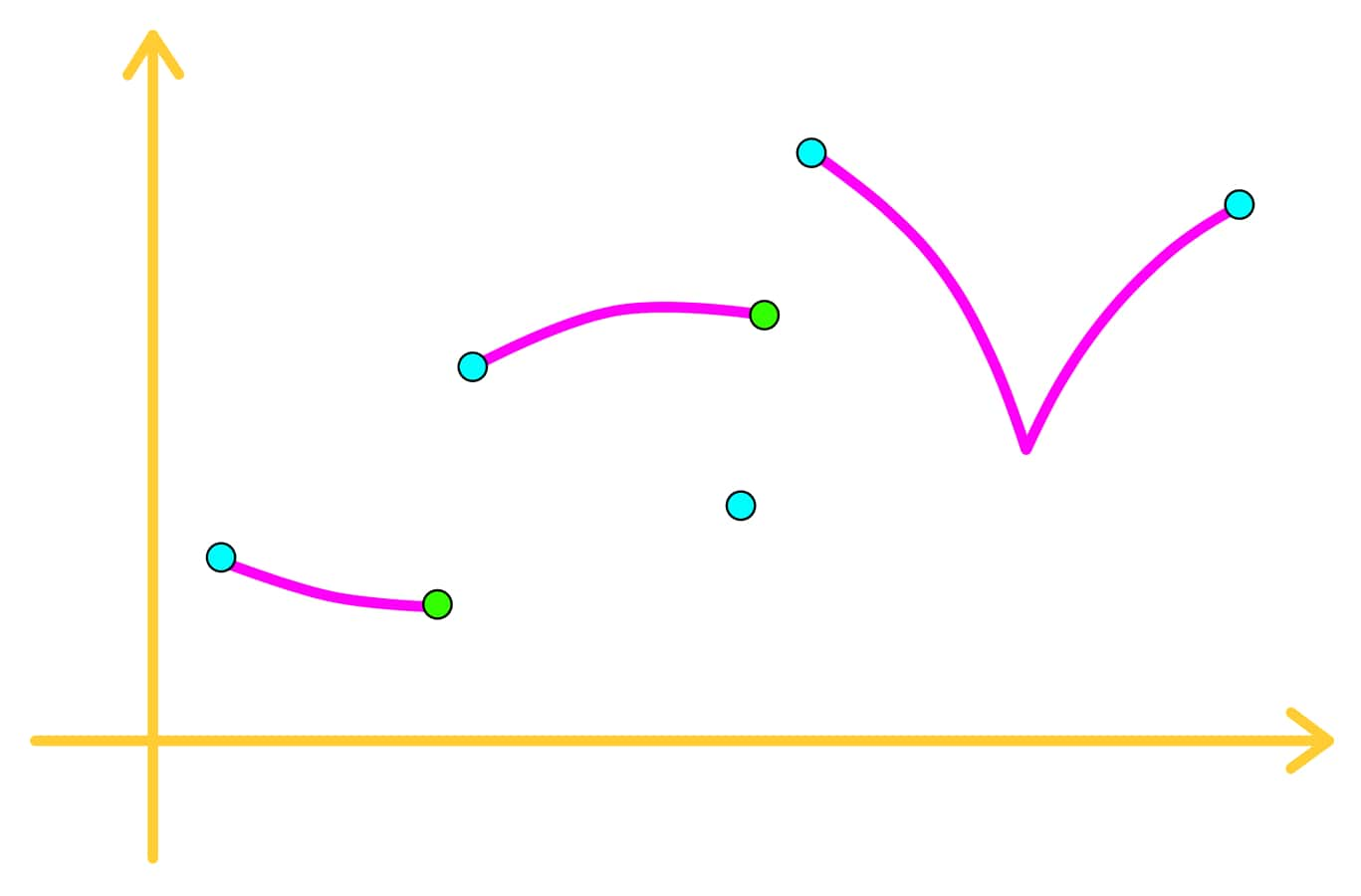# Introduction to the laplace transform

## What is a Laplace transform?

The Laplace transform is a particular integral formula which is very useful to solve linear differential equations with certain conditions. The reason why you will prefer to use the Laplace transformation instead of any other method while solving certain linear differential equations directly, is because this method actually simplifies the process by being algebra-based instead of calculus-based.

In mathematics, we refer to a "transformation" as a method we use to simplify the way we solve a problem, sometimes this means going from the techniques used in a higher level of math to a lower level of the field. In this case, the integral transform which we sometimes might refer as the Laplace integral, is a tool to convert a linear differential equation which is a function of time into an algebraic expression in terms of frequency.

In mathematical notation, the definition of Laplace transform or Laplace integral can be simply put as follows:Formula 1. Laplace transform for a function f(t)f(t)f(t) where t≥0\;t \geq 0t≥0.

As you may have noted in formula 1, we are dealing with an improper integral in the Laplace transform since the interval of the integration is unbounded. Remember that when one (or both) of the extremes of an integration is infinite we truly do not know the value itself (because is not a real number) and so, we cannot evaluate the integration using the classical Riemann technique.

From our lessons on limits you may remember that you can know the behaviour of a function even though you may not know its value by evaluating its limit as it approaches a particular point. We will use this knowledge when solving improper integrals.

To take the issue of the unbounded interval in the integration out, we take the limit of the integration giving the unbounded side of the integral a constant value, which will approach infinity in the limit operation. In this way, we can easily use regular integration techniques to solve the problem without being worried to evaluate to infinity until we are able to toss away those variables. In simpler words, we want to solve the integration in a way that we get rid of all the t's of the function, so we don't have to worry about evaluating it to infinite, and thus, simplifying the problem by now having the function in other terms.

The importance of the Laplace transform will be more obvious to us if we think on the whole process of solving a differential equation with it. A Laplace transform is an operator, this operator will be applied to a differential equation that in principle is difficult to solve for, so the steps go as follows:

First you have a differential equation to which you apply the operator called the Laplace integral (figure 1), this will produce an algebraic expression which is much simpler to solve and obtain a result. After obtaining the information needed from the first equation (say the value of a variable, etc) we can even work backwards to check if our process (and result) is correct, thus giving us a complete cycle of information. For this last step we use the operator called the inverse Laplace transform, and we will dedicate a complete article on it later.

## Laplace transform examples:

So now to the important question, how do we actually solve laplace transforms? In this section we will be looking into the Laplace transformations of the most common functions.

1. Laplace transform of 1

A great example of a function which value is 1 on the unbounded interval from zero to positive infinity is called the unit step function. The unit step function, or heaviside step function is a discontinuous functions which has a value of zero for all of its points on the negative quadrant, and a value of 1 for all of its points in the positive quadrant.

Taking the Laplace transform of f(t) = 1

Now we evaluate the limit:

So the Laplace transform of the unit step function is equal to 1/s or: $F(s)=\frac{1}{s}$

Something imperative to understand is that for this example we are working under the assumption that s is a positive number in order forIf we were to change s to having a negative value this would change the sign of the exponent to positive and therefore we would end up with an infinite limit in that part of the calculation, so to finish with this example we say that the Laplace transformation of the step unit function is equal to 1/s under the condition that s is positive (bigger than zero).

After seeing this last calculation is important to mention that the same process would apply for any Laplace transform of constant value functions on the interval from zero to infinity since a constant value would end up factorized out of the integration and multiplied to this same result.

Having any doubts? Check for yourself while you practice solving the next example:

Obtain the Laplace transformation of the function f(t)=5 for the interval from 0 to infinity.

2. Laplace transform of t

Having the function $f(t)=t$ this is what we call the ramp function, and it looks like this:

• We take the Laplace transformation:
• In this case let us integrate by parts the integration without interval first, and then we will plug it back into the transformation:
• Therefore the Laplace transformation continues as follows:
• As we evaluate the limit of the function as c goes to infinity, the exponentials containing c terms go to zero, since the exponent is negative as long as s is positive, therefore:

3. Laplace transform of exponential function

Now let us look into an example where the function we will be computing the Laplace transformation to is an exponential. So for this case we have that $f(t)=e^{at}$

• Therefore, if we apply the Laplace transform to it we end up with the next operation:
Notice how we have simplified the equation by using the rules of exponents. Since the integration is being taken just with respect to t, then we can treat a and s as constants, and so (a-s) is taken as a single constant itself.
• Now, to solve the transformation, remember we have an improper integral so we need to be taking the limit of the integration in order to evaluate it as t is approaching infinity:
Which simplifies to:
• In this case, we pay attention to the constant (a-s) again, because depending on the size of a and s relative to each other we can have different answers. Next we have the three possible cases:

When a>s, (a-s) is a positive number:

the limit goes to infinity and so, it doesn't exist.

When a=s, (a-s) is zero:

When a < s, (a-s) is a negative number: (we will call (a-s)=-k for simplification purposes)

So as you can see, for the Laplace transform of an exponential, the limit exists only when s > a

4. Laplace transform of sine

For this section we have the function $f(t)=\sin (wt)$
• Let us solve the integral part using integration by parts:
From this notice that the first part of the solution goes to zero:
• So we are left with:
• And once more, we solve using integration by parts
Where the first part of the result simplifies to:
• So the integral looks as follows:

So we have gone as far as we want to go for the solution of this Laplace transform, if you notice in here you have gotten back to the beginning integration we wanted to solve for, so you may be wondering: are we just going to go in circles?

Fast answer: NO, it actually help us out to solve it right away, and so we go back to our very first equation and equal it to the result we have gotten so far:

Or simply put:
• Let us now use simple algebra to solve this problem:
• Therefore:
• So:

For all of the examples we have seen in this article, make sure to check out our videos at the top on the Introduction to the Laplace transform. Also make sure to check the sections on Calculating Laplace transforms and Solving differential equations with the Laplace transform.

## Laplace transform of piecewise function:

Remember that a piecewise function refers to a function that is broken in pieces or sub-functions. For us to take the Laplace transform of a piecewise function this needs to be continuous on each sub-function (or interval) we are applying our transform to. Each interval of the function will have a different value, therefore we have to break down our Laplace integration into as many integrals as pieces of the function we have. This may actually simplify our laplace integration in certain sections of the piecewise function,since whichever section is not unbounded (that is, which falls in an interval where none of the extremes is infinite) can be solved by regular integration steps.

The most important factor to keep in mind while working with piecewise functions is that you need to remember all the pieces you are integrating, make sure that at every step of the process you have an integration (or a result of an integration) for each of the continuous pieces of the function.

Example:

The piecewise function has two pieces, therefore, our Laplace transformation will consist of two integrations:

## Wrapping up on Laplace transforms:

In conclusion, the Laplace transform is a good operator to be applied to certain types of differential equations to simplify them into algebraic expressions. Although in this article we have gone step by step on the solution for the most common functions this has been mostly for educational purposes, usually when solving complicated differential equations through Laplace transforms we just have a little table of the most basic function solutions at hand, this allows us to solve for functions which happen to be a combination of the ones shown here.

Something to remember is that for Laplace transformations we can always factorize out constants or apply the transform distributedly whenever we have a function involving addition or subtraction, in other words, if we have a function with terms in the form f(t)=A(t) + B(t), we can simply apply the Laplace transform to A and B separately and then add the results at the end.

You will get practice at doing this and other tricks as you continue to nurture your skills solving differential equations, for now, I leave you with a table containing the solution for the most common functions, use it as a reference, but remember, if you do not understand the results you can always go back to this article and check them up.

For additional examples on these subject, we recommend you to take a look at these Differential Equations notes, where you can find a section dedicated to the Laplace transform, its definition and examples.

### Introduction to the laplace transform

#### Lessons

Continuity:

A function is called piecewise continuous on an interval if it can be broken into a finite number of subintervals that are all continuous.Is an example of a function that is piecewise continuous.

Definition of the Laplace Transform:

If $f$($t$) is a piecewise continuous function, then the Laplace Transform of $f$($t$) denoted as $L${$f$($t$)} is defined as,

$L${$f$($t$)} = $\int_{0}^{\infty}e^{-st}f(t) dt = F(s)$

• Introduction
What are Piecewise Continuous Functions, and what is the Laplace Transform?

• 1.
Determining Laplace Transforms

What is the Laplace Transform of $f$($t$) = 1? Or in other notation what is $L${1}?

• 2.
What is the Laplace Transform of $f$($t$) = $e^{at}$? Or in other notation what is $L${$e^{at}$}?

• 3.
What is the Laplace Transform of $f$($t$) = $t$? Or in other notation what is $L${$t$}?

• 4.
What is the Laplace Transform of $f$($t$) = $\sin(\omega t)$? Or in other notation what is $L${$\sin(\omega t)$}?